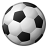# QlikView App Dev

Discussion Board for collaboration related to QlikView App Development.

cancel
Showing results for
Did you mean:Not applicable

## Set Analysis, restriction to ALL, TOTAL, \$, {1}

Dear Fellows,

I'm strugling with following task. Maybe I didn't get correct the difference between ALL, TOTAL, \$, {1} in set analysis.

My goal is to redistribute values from one material (99) on many another (M1 and M2).

It seems my solution works almost correct, however without applying any filter on specific material.

###### Here is my formula and many another tries. *<vBonusSplit> helps easily maintain parameters on which subtotal should be created the sum. You can delete for example "Variant" and see the result will change.

 =sum(total<\$(vBonusSplit)>Rebate)*sum(Sales)/sum(total<\$(vBonusSplit)>Sales)

I've tried to modify this formula with all, {1} and \$ but without any success.

After I apply a filter on specific material, redistributed values (in Rebate on Position column) disappear.

How to resolve this issue to keep always the values right in the according row?

Thank you in advance for your hints, which could be helpful.

Regards

Stan

1 Solution

Accepted SolutionsCreator

Hi Stan,

"All" disregards selections, and, if used, in chart, dimensions (ALL field) is the same as  ({1} total field)

"Total" does not disregard selections, but it disregards dimensions.

Please see the below link, for the difference between ALL, TOTAL

http://community.qlik.com/message/336161#336161

In your case, try changing your 'Rebate on POSITION' expression to either of the following,

this will make it work irrespective of the selections:

=sum({1} TOTAL <\$(vBonusSplit)>Rebate)

*sum(Sales)

/sum({1} TOTAL <\$(vBonusSplit)>Sales)

or

=sum(ALL <\$(vBonusSplit)>Rebate)

*sum(Sales)

/sum(ALL <\$(vBonusSplit)>Sales)

HTH

KD

4 RepliesCreator

Hi Stan,

"All" disregards selections, and, if used, in chart, dimensions (ALL field) is the same as  ({1} total field)

"Total" does not disregard selections, but it disregards dimensions.

Please see the below link, for the difference between ALL, TOTAL

http://community.qlik.com/message/336161#336161

In your case, try changing your 'Rebate on POSITION' expression to either of the following,

this will make it work irrespective of the selections:

=sum({1} TOTAL <\$(vBonusSplit)>Rebate)

*sum(Sales)

/sum({1} TOTAL <\$(vBonusSplit)>Sales)

or

=sum(ALL <\$(vBonusSplit)>Rebate)

*sum(Sales)

/sum(ALL <\$(vBonusSplit)>Sales)

HTH

KDPartner - Creator III

do you mean this?Partner - Creator III

ah now i got it. thats your expression

=sum(total<\$(vBonusSplit)> {<Material=>} Rebate) // /XRate

*sum( {<Material=>} Sales) // /XRate

/sum(total<\$(vBonusSplit)> {<Material=>} Sales) // /XRateNot applicable
Author

Thank you very much, KedarDan,

indeed this is what I was looking for. Both of the formulas are working, I decided to use the first formula:

=sum({1} TOTAL <\$(vBonusSplit)>Rebate)

*sum(Sales)

/sum({1} TOTAL <\$(vBonusSplit)>Sales)

You have been a great help.

Regards

Stan